# Why `irate from Prometheus doesn't capture spikes`

Prometheus query language (PromQL) has two similar functions for calculating per-second rate over counters such as `requests_total` or `bytes_total`rate and irate. There is a myth about `irate` function — it captures per-second rate spikes on the given `[range]`, while `rate` averages these spikes.

# Spikes and irate

Look at the following picture for hypothetical `requests_total` counter:

It contains values `[20,50,100,200,201,230]` with timestamps `[10,20,30,40,50,60]`. Let’s calculate `irate(requests_total[40s])` at the point `t`. It is calculated as `dv/dt` for the last two points before `t` according to the documentation:

`(201–200) / (50–40) = 0.1 rps`

The `40s` range ending at `t` contains other per-second rates:

• `(100–50) / (30–20) = 5 rps`
• `(200–100) / (40–30) = 10 rps`

These rates are much larger than the captured rate at `t`. `irate` captures only 0.1 rps while skipping 5 and 10 rps. Obviously `irate` doesn’t capture spikes. Irate documentation says:

`irate` should only be used when graphing volatile, fast-moving counters.

It is expected to capture spikes for volatile, fast-moving counters. But `irate` returns a sample of per-second rates for such counters. The returned sample may contain all the spikes, a part of spikes or it may miss all the spikes and capture random rates. This highly depends on the following query_range API args: `start` and `end` values (i.e. graph time range) and the `step` value (i.e. graph resolution and zoom level). This means that the graph built with `irate` tends to jump in arbitrary directions during zooming and scrolling. This is especially visible on big `step` values covering multiple time series points (aka multiple scrape intervals).

The following graphs are captured for the same query — `irate(requests_total[25m])` on the same time range. The only difference is modified `step` in Grafana from `20m` to `21m`.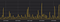irate(requests_total[25m]), step=20m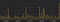irate(requests_total[25m]), step=21m

As you can see, these graphs look completely different and they definitely don’t catch spikes.

Let’s add green `rate` line to these graphs: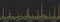green line — rate(requests_total[25m]), step=20m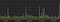green line — rate(requests_total[25m]), step=21m

Green `rate` line is much more consistent on these graphs comparing to yellow `irate` line for the same counter.

# Capturing spikes

Previous graphs revealed that both `irate` and `rate` don’t capture peaks on rapidly changing counters. Are there approaches for capturing spikes with PromQL? Probably, recently added subqueries could be used somehow, but I couldn’t figure out how to do it reliably.

If you still want capturing spikes on volatile counters, then set up VictoriaMetrics as a remote storage for Prometheus and then query VictoriaMetrics with `rollup_rate` function from PromQL extensions. This function returns `min`, `avg` and `max` values for per-second rate. The rate is calculated for each adjacent points, so spikes are reliably captured in `min` and `max` values, while `avg` value is usually close to `rate` value, though it is calculated differently.

The following graph contains `min` and `avg` values for `rollup_rate`: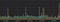rollup_rate(requests_count), step=21m, without rollup=”max”

`rollup=”min”` is red, while `rollup=”avg”` is blue. Yellow line is for `irate`. As you can see, red line reliably captures all the minimum rates, while yellow line only sometimes reaches the the actual minimum rates.

Now let’s look at the graph with `rollup=”max”`. It has bigger vertical scale, since rate spikes are much higher for the `requests_rate` counter comparing to the average rate: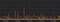rollup_rate(requests_count), step=21m, with rollup=”max”

All the lines from the previous graph are present here for comparison. As in the previous case, yellow line (`irate`) only sometimes reaches actual maximum rates (spikes).

# Conclusion

`Irate` doesn’t capture spikes — it just returns a sample of per-second rate values. If you want to capture all the spikes on volatile counters, then use `rollup_rate` function from VictoriaMetrics’ PromQL extensions.

There is another widespread myth about `irate` — it is a faster alternative to `rate`. The origin of the myth is: `irate` takes only two last points on the given `[range]` interval, while `rate` requires all the points on the `[range]` interval. While this is true, the performance difference is usually negligible, since Prometheus spends CPU time on extracting all the time series points for the given `[start … end]` interval of the query_range API regardless of the used function.

If in doubt, prefer `rate` over `irate`, since `rate` consistently returns average per-second rate values for the given `[range]`, while `irate` usually returns a random set of per-second rate values, which may look like garbage for volatile fast-moving counters.

Update: Chris Siebenmann wrote an interesting article on how to capture spikes and dips in Prometheus with irate + subqueries.

Update2: VictoriaMetrics is open source now, so you can investigate how it implements `rollup*` functions.

Founder and core developer at VictoriaMetrics

## More from Aliaksandr Valialkin

Founder and core developer at VictoriaMetrics